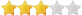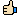知識庫W, R, X, 711, 755, 777, 666 是什麼意思？ 作者 iSmile Helpdesk 於 01/02/2006 08:00 AM 讀取 Read = R = 4 寫入 Write = W = 2 執行 eXecute = X = 1 Owner = 程式擁有人 Group = 同一群組的其它帳號 Other = 全世界，如訪客 (1) 如果您要開放檔案給任何人 "讀取"、並能 "寫入"，那麼： Owner (程式擁有人) = 4 + 2 + 0 = 6 Group (同一群組) = 4 + 2 + 0 = 6 Other (訪客) = 4 + 2 + 0 = 6 所以 Owner Group Other = 666 (2) 如果您要開放檔案給任何人執行，自己可以更改檔案，但是不希望其它人更改您的檔案，那麼： Owner (程式擁有人) = 4 + 2 + 1 = 7 Group (同一群組) = 4 + 0 + 1 = 5 Other (訪客) = 4 + 0 + 1 = 5 所以 Owner Group Other = 755 [*] 正確的權限請參考您程式的安裝說明。 [*] 權限 777 是非常危險的設定。表示任何人都可以修改您的程式，然後執行。不但危害您帳號內的資料，也容易將您的帳號轉化為攻擊他人的跳板！(1914 投票數)有幫助無幫助

 完整姓名： 電子郵件： 評論：